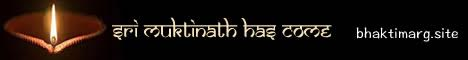Note:  Do not rely on this information. It is very old.

# Units

Units are the standards of reference in terms of which we measure any concrete quantity. Thus we may measure a length in feet or metres, in which case the foot or the metre is the unit of length. That such standards should be absolutely fixed is obviously necessary. Although in olden days many pounds were in use, and each pound had its name, e.g. the tower pound, the merchants pound, etc., and indicated a definite mass, nevertheless it is extremely probable that the existence of many different standards made it more difficult for the ignorant to avoid being the victims of commercial deception. In modern times the tendency has been to reduce the number of units as far as possible -- to have, in fact, only one unit for each kind of quantity. We in England, however, are far from perfect in this respect, as is justified by the existence of the pounds troy and avoirdupois as units of mass, while area on a small scale is given in square feet at the same time that the field is measured in acres. A gallon and a cubic foot are both units of volume, but memory must play its part in connecting one or the other, as well as in the reduction of acres to square feet. This kind of multiplicity of units arises from the fact that our whole system is perfectly arbitrary and wholly unscientific. There is no connection between our common units of volume or area and that of length. A scientific system, on the other hand, chooses its unit of area to be the square of its unit of length, and its unit of volume to be the cube of the same. This simple state of things exists in the centimetre, gram, second, or C.G.S. system, and it is therefore adopted in scientific work all over the earth. In it the centimetre (equal to .394 of an inch) is the unit of length, the square centimetre that of area, while volume is estimated in cubic centimetres. The unit of mass is the gramme, that being the mass of a cubic centimetre of pure water at the temperature at which it has its maximum density (4° C.); and the unit of time is the second. Again, instead of having to execute a series of mental gymnastics, such as are required and transforming a certain number of, say, ounces and tons or inches into furlongs, all such changes are made by means of a multiplication or division by powers of ten. The so-called "dry measure" has no analogy in a scientific system, so that one is spared the inconvenience of endeavoring to realize what is meant by, say, a chaldron of coke, even if one does succeed in reducing it to bushels, since such a substance can only be reasonably estimated by weight. A unit, such as that of length or volume, which is defined by reference to another unit -- in this case, that a length -- is termed a derived unit.